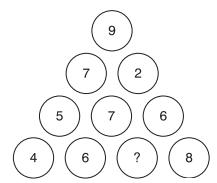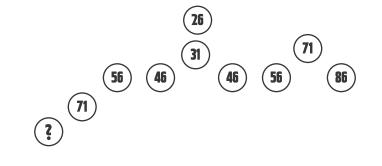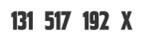﻿ Number Puzzles with Answers | Number Puzzles for Interviews | Number Puzzles Questions Answers 2013 | Number Puzzles Quiz Questions and Answers Pdf 2013 | Number Series Puzzles Questions Answers | Number Puzzles Interview Questions Answers | Cross Number Puzzles | Number Puzzles for Math Geniuses | Brain Puzzle Questions |EnggRoom
Asp.Net Project
Vb.Net Project
Php Project
Java Project
C/C++ Project
Android Project
Others Project
Latest Seminar
Study Material
Placement Paper
Company Placement Paper
Gate Paper
Miscellneous
Best Project Ideas
Interviews Questions For Freshers
Request
Feedback

Puzzle 1:- Complete the Following SequenceAns:- 6,561.
Sol:-There are two sequences arranged alternately. In each sequence the number is the square of the previous number in that sequence. 6,561 is the square of 81.

Puzzle 2:- Which Number is Odd Man Out?Ans:- 4275.
Sol:-The number formed by the first two digits plus the number formed by the second two digits is 99.Say For Example:-3663 is formed as = 36+63=99

Puzzle 3:- What number should replace the question mark?Ans:- 86.

Puzzle 4:- What number should replace the question mark?Ans:- 7.

Sol:-The numbers in each line contain the digits 1 – 9 only once.

Puzzle 5:- What number should replace the X?Ans:- 1 (One).

Sol:-Space the Seriese Correctly.Seriese will become 13,15,17,19,2(1).